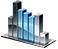Operators and Expressions

# Operators and Expressions

## Arithmetic operators

 Addition of values: a=b+c; Subtraction of values: a=b-c; Multiplication of values: a=b*c; Division of values: a=b/c; Remainder of a division: a=b%c; Sign change: a=-a; Increment: a++ Decrement: a--

## String operators

 String concatenation a=b+c;

## Assignment operators

 Assigning the A value to the variable B B = A; The sum of A and B is assigned to the variable А A += B; The difference between the values A and B is assigned to the variable А A -= B; The product of the values A and B is assigned to the variable A A *= B; Quotient from the division of A into B is assigned to the variable A A /= B; The bitwise operation of A AND B with the result assignment to A A &= B; The bitwise operation A OR B with the result assignment to A A |= B; The bitwise operation exclusive OR between A and B, with the result assignment to A A ^= B;

## Logical and relational operators

 TRUE if B equals to A B == A; TRUE if B does not equal to А B != A; TRUE if B is less than A B < A; TRUE if B is less that or equal to A B <= A; TRUE if B is greater than A B > A; TRUE if B is greater than or equal to A B >= A; TRUE if A or B is equal to TRUE A || B; TRUE if both A and B are equal to TRUE A && B;

## Bitwise operators

 Bitwise NOT ~A; Shifting the binary representation of B to A bits to the right B >> A; Shifting the binary representation of B to A bits to the right (the bit sign does not change) B >>> A; Shifting the binary representation of B to A bits to the left B << A; Bitwise OR for the A and B numbers A|B; Bitwise AND for the A and B numbers A&B; Exclusive OR for the A and B numbers A^B;

## Operators precedence

The following table shows the precedence of NetTradeX language operators. Operators with the highest precedence appear at the top of the table.

 * / % Multiplication, division, and modulus + - Addition and subtraction << >> Bit-shift & Bitwise AND ^ Bitwise exclusive OR | Bitwise OR <= < >= > Comparison operations == != Equality/inequality operations && Logical AND || Logical OR ?: Ternary operation = += -= *= /= %= &= |= ^= <<= >>= Assignment